# Lenses

GCSEAQAPhysics FoundationPhysics Higher

## Lenses

Lenses change images by refracting light. Ray diagrams are used to represent the path of light through lenses and can be used to show how images are formed through a lens. Lenses can be either concave or convex

## Convex Lenses

Convex lenses are thicker in the middle than at the edges. Light entering the convex lens is refracted inwards towards a point called the principal focus. There is a principal focus on either side of the lens, for rays entering the lens from each direction. The distance between the centre of the lens to the principal focus is called the focal length. The ray diagram below shows the effect of a convex lens on the path of light rays.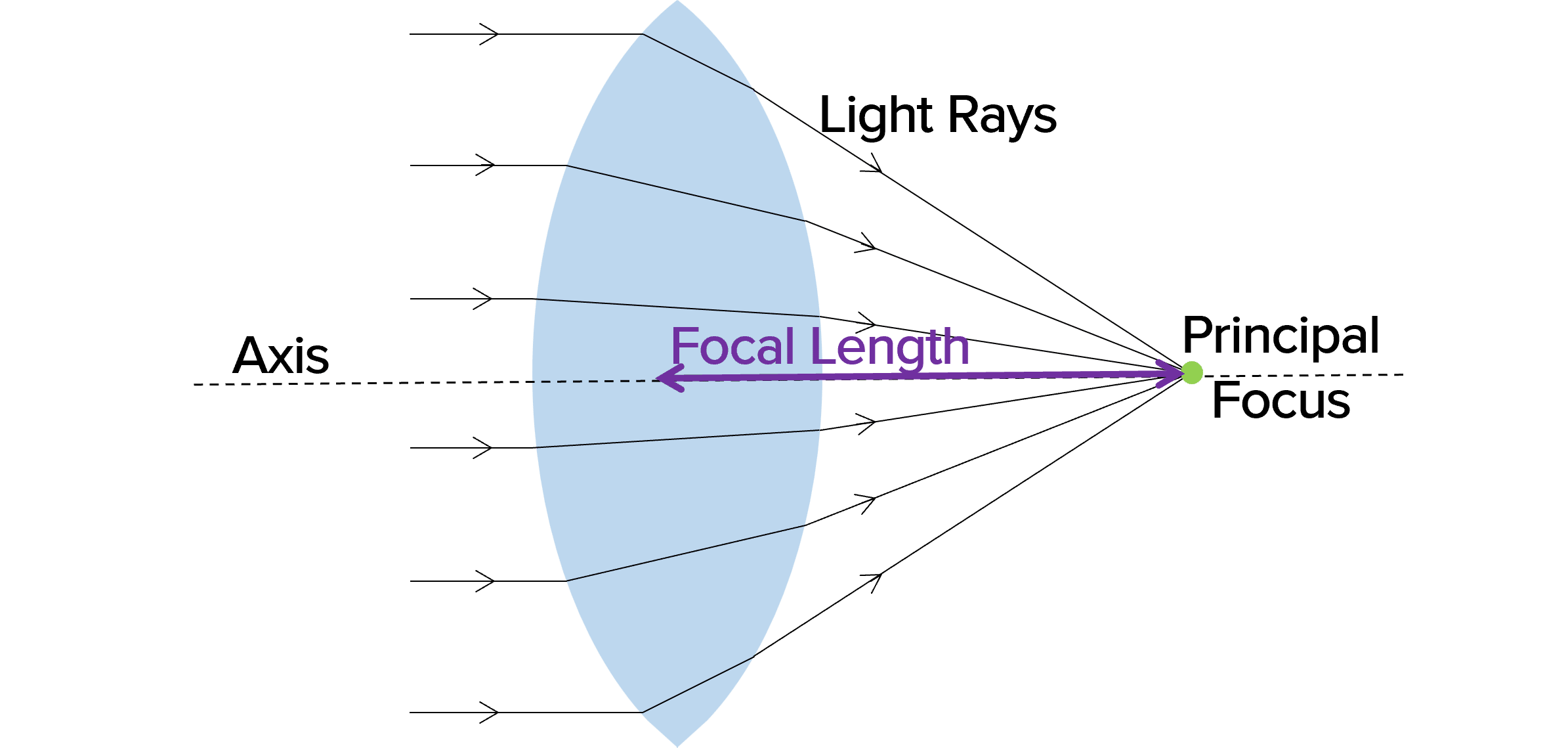You need to remember the following rules about convex lenses:

• Rule 1: Any ray that enters the lens parallel to the axis will refract through the lens and pass thorough the principal focus.
• Rule 2: Any ray that enters the lens from the principal focus will refract through the lens and leave the lens parallel to the axis
• Rule 3: Any ray that enters the lens along the axis will continue in the same direction.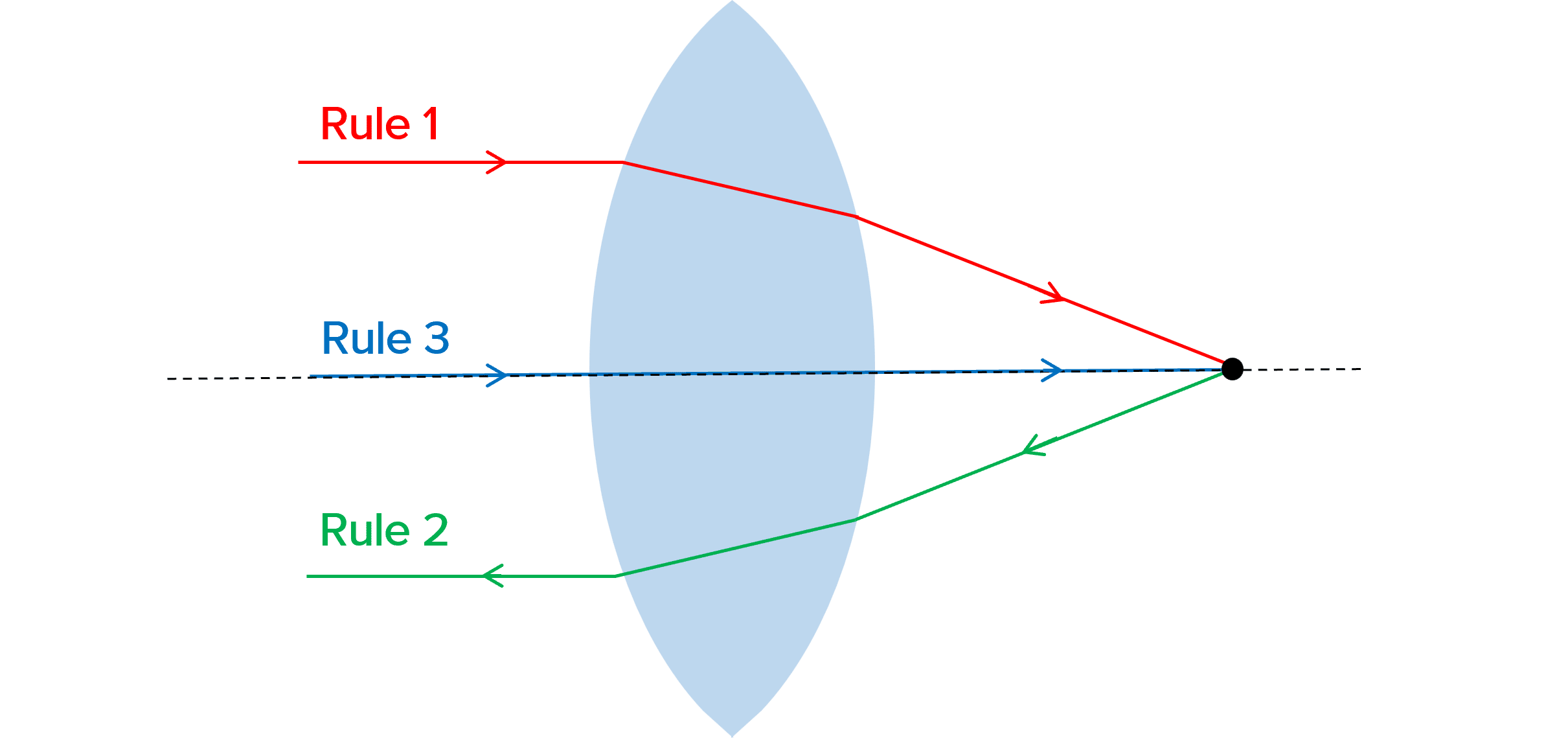GCSEPhysics FoundationPhysics HigherAQA

## Concave Lenses

Concave lenses are thicker at the edges and thinner in the centre. They cause light to diverge, meaning spread out. The principal focus of a concave lens is the point where rays hitting the lens parallel to the axis appear to have come from. We represent this on a ray diagram by tracing virtual rays backwards from the ray leaving the lens. Like a convex lens, there is a principal focus on both sides of the lens.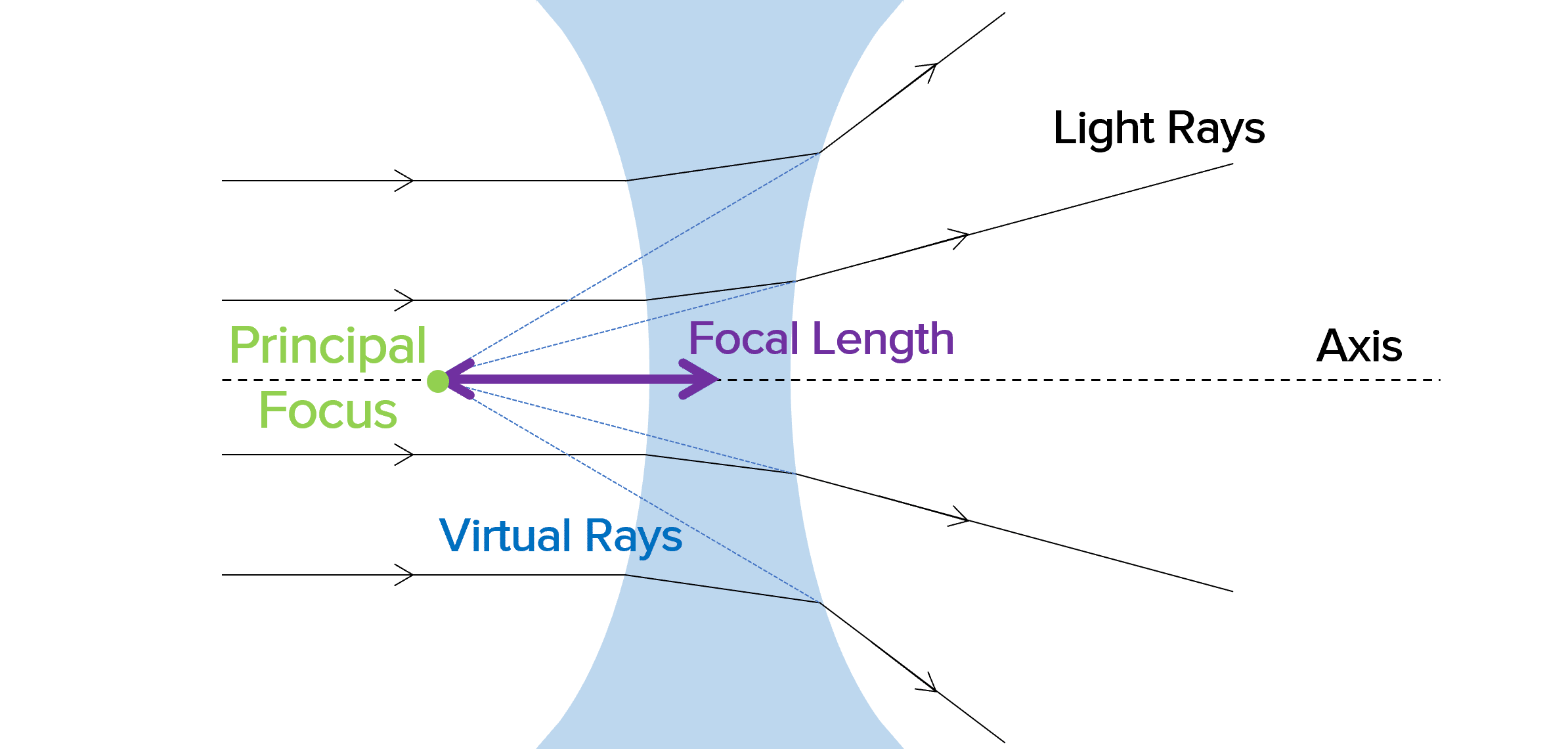There are also rules to remember for concave lenses:

• Rule 1: Any ray that enters the lens parallel to the axis will refract through the lens and travel in line with the principal focus so that it appears to have come from the principal focus.
• Rule 2: Any ray that enters the lens, travelling towards the principal focus refracts through the lens and leaves it parallel to the axis
• Rule 3: Any ray that enters the lens along the axis continues in the same direction.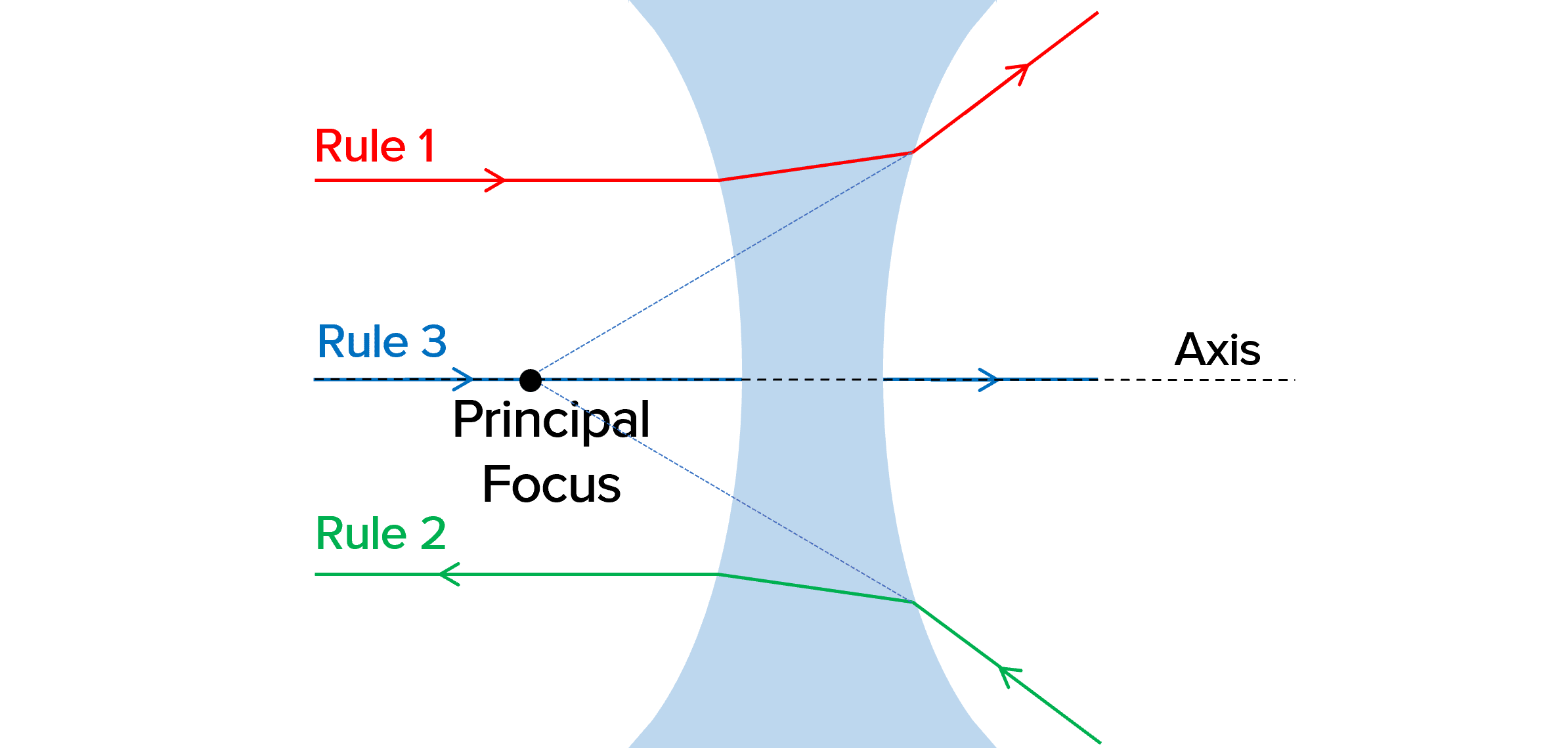GCSEPhysics FoundationPhysics HigherAQA

## Real and Virtual Images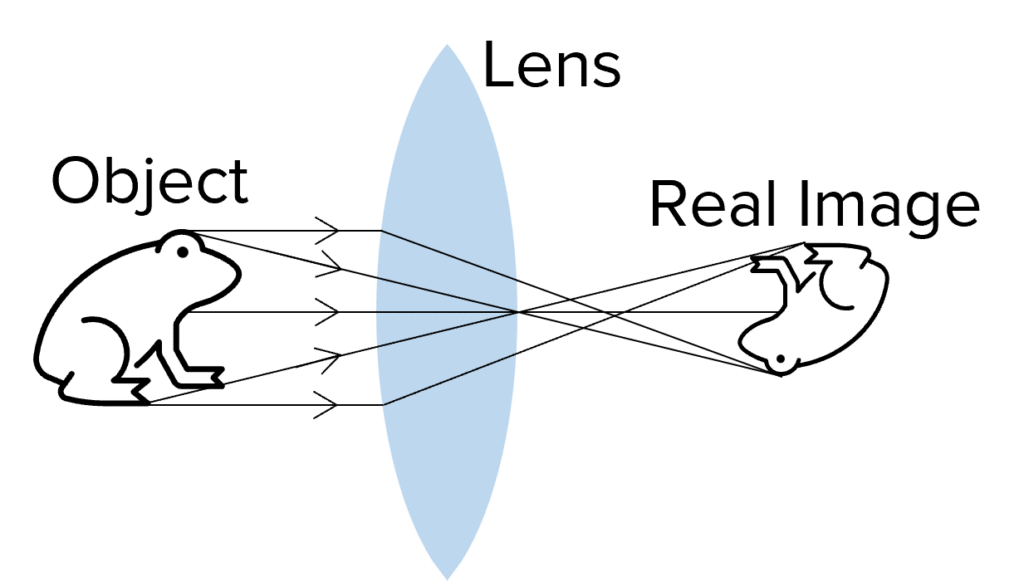When light comes together to form an image, such as on a screen or on the retina (the back of the eye), a real image is formed.

Only convex lenses produce real images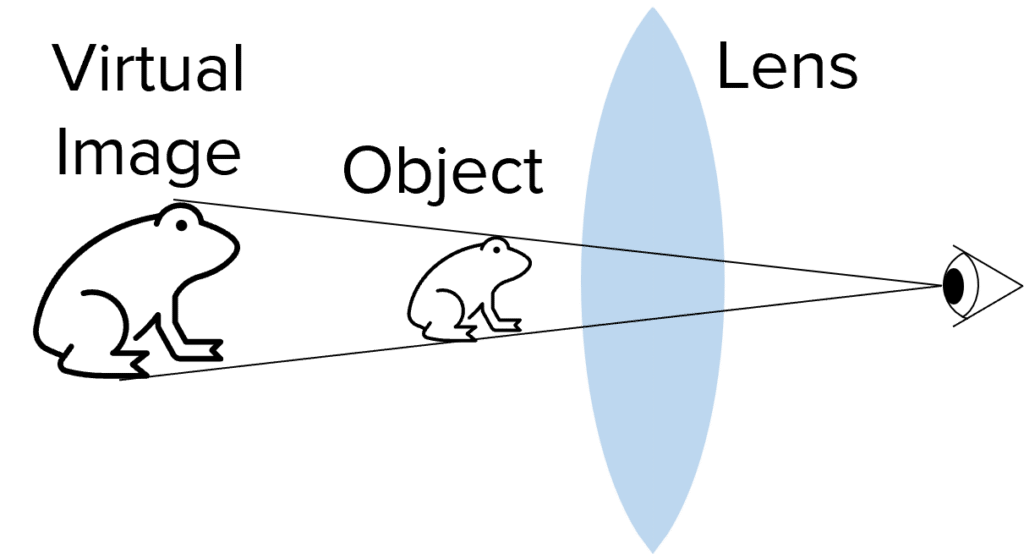A virtual image is formed when light rays are diverging, and so the object appears to be in a different place. For example, when you look into a mirror, you see a virtual image. This is because the reflection appears to be behind the mirror.

Concave lenses always produce virtual images. Convex lenses may also produce virtual images.

GCSEPhysics FoundationPhysics HigherAQA

## Magnification

When an object is magnified, a virtual image of the object is created that is larger than the object itself. The image is called a magnified virtual image.

For magnification to occur, the object must be closer to the lens than the principal focus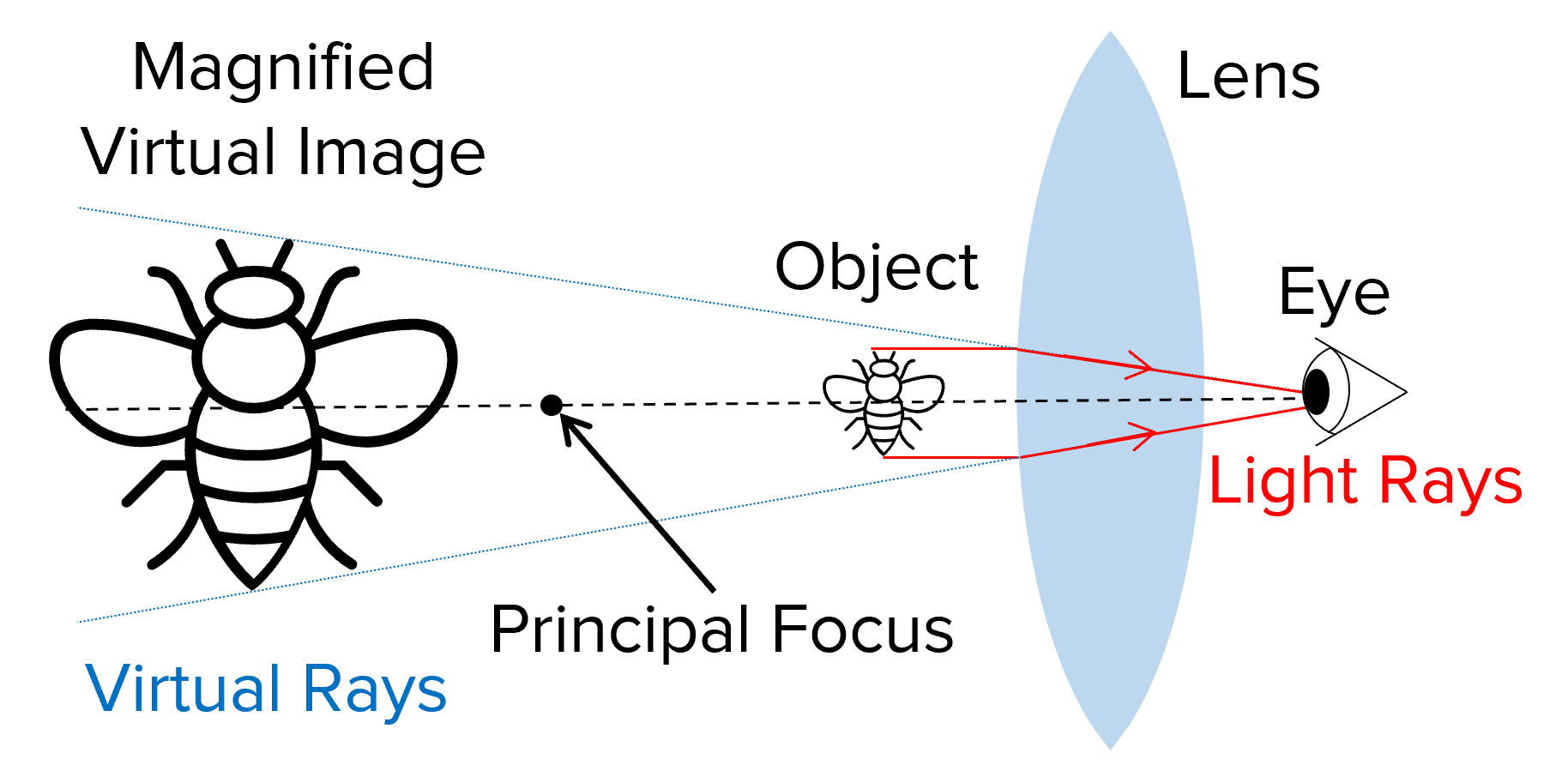You can calculate how much the image has been magnified (increased size) using the following formula:

$\text{magnification}=\dfrac{\text{image size}}{\text{object size}}$

Magnification is a ratio and so it has no units

If a virtual image has a negative magnification, this means the image is smaller than the object.

GCSEPhysics FoundationPhysics HigherAQA

## Example: Calculating Image Size Using Magnification

A flower is measured to be $3\text{ cm}$ tall. A magnifying glass with magnification of $4$ is used to view the flower. How tall does the flower appear to be?

[2 marks]

Magnification equation:

$\textbf{magnification}=\dfrac{\textbf{image size}}{\textbf{object size}}$

Rearrange to find the image size:

$\text{image size}=\text{magnification} \times \text{object size} = \textcolor{aa57ff}{4} \times \textcolor{f21cc2}{3} \text{ cm} = \bold{12} \textbf{ cm}$

GCSEPhysics FoundationPhysics HigherAQA

## Lenses Example Questions

Ray diagram.Gold Standard Education

A convex lens is thicker at the centre/bulges out in the middle.

And thinner at the edgesGold Standard Education

a. False.

Any ray that enters a convex lens along the axis will continue in the same direction.

b. True.

c. True.Gold Standard Education

A real image is formed when light comes together to form an image.

A virtual image is formed when light rays are diverging, and so the object appears to be in a different place.Gold Standard Education

$5 \text{ mm} = \bold{0.5} \textbf{ cm}$ \\ \begin{aligned} \textbf{magnification} &= \dfrac{\textbf{image size}}{\textbf{object size}} \\ &= \dfrac{6}{0.5} \\ &= \bold{12} \end{aligned}Gold Standard Education

## Lenses Worksheet and Example Questions

GCSEOfficial MME

### Electromagnetic Waves Questions 2

GCSEOfficial MME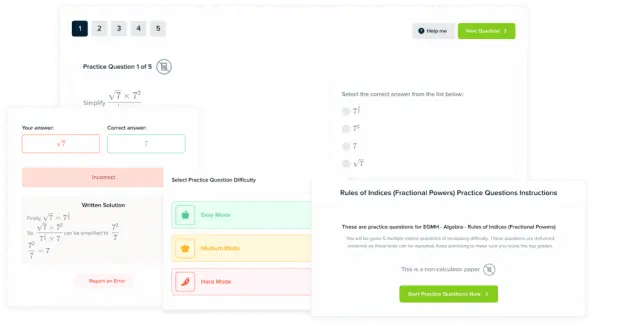Product